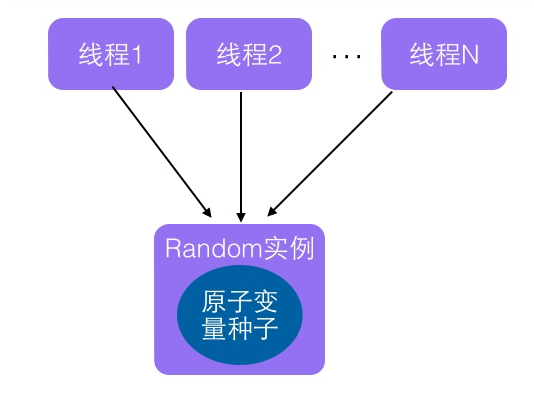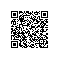# 二、 Random类原理及其局限性

public class RandomTest {
public static void main(String[] args) {

//(1)创建一个默认种子的随机数生成器
Random random = new Random();
//(2)输出10个在0-5（包含0，不包含5）之间的随机数
for (int i = 0; i < 10; ++i) {
System.out.println(random.nextInt(5));
}
}
}
• 代码（1）创建一个默认随机数生成器，使用默认的种子。
• 代码（2）输出输出10个在0-5（包含0，不包含5）之间的随机数。

    public int nextInt(int bound) {
//(3)参数检查
if (bound <= 0)
//(4)根据老的种子生成新的种子
int r = next(31);
//(5)根据新的种子计算随机数
...
return r;
} 

• 首先需要根据老的种子计算生成新的种子。
• 然后根据新的种子和bound变量通过一定的算法来计算新的随机数。

    protected int next(int bits) {
long oldseed, nextseed;
AtomicLong seed = this.seed;
do {
//(6)获取当前原子变量种子的值
oldseed = seed.get();
//(7)根据当前种子值计算新的种子
//(8)使用新种子替换老的种子
} while (!seed.compareAndSet(oldseed, nextseed));
//(9)
return (int)(nextseed >>> (48 - bits));
}
• 代码（6）使用原子变量的get方法获取当前原子变量种子的值
• 代码（7）根据具体的算法使用当前种子值计算新的种子
• 代码（8）使用CAS操作，使用新的种子去更新老的种子，多线程下可能多个线程都同时执行到了代码（6）那么可能多个线程都拿到的当前种子的值是同一个，然后执行步骤（7）计算的新种子也都是一样的，但是步骤（8）的CAS操作会保证只有一个线程可以更新老的种子为新的，失败的线程会通过循环从新获取更新后的种子作为当前种子去计算老的种子，可见这里解决了上面提到的问题，也就保证了随机数的随机性。
• 代码（9）则使用固定算法根据新的种子计算随机数，并返回。

public class RandomTest {

public static void main(String[] args) {
//(10)获取一个随机数生成器

//(11)输出10个在0-5（包含0，不包含5）之间的随机数
for (int i = 0; i < 10; ++i) {
System.out.println(random.nextInt(5));
}
}
}private static final AtomicLong seeder = new      AtomicLong(initialSeed());

private static long initialSeed() {

//获取变量java.util.secureRandomSeed的值
String pp = java.security.AccessController.doPrivileged(
new sun.security.action.GetPropertyAction(
"java.util.secureRandomSeed"));
//如果变量值为true则使用安全性较高的种子，这个种子是不可被预测的
if (pp != null && pp.equalsIgnoreCase("true")) {
byte[] seedBytes = java.security.SecureRandom.getSeed(8);
long s = (long)(seedBytes) & 0xffL;
for (int i = 1; i < 8; ++i)
s = (s << 8) | ((long)(seedBytes[i]) & 0xffL);
return s;
}
//否者使用当前时间来计算初始化种子
return (mix64(System.currentTimeMillis()) ^
mix64(System.nanoTime()));
}

private static final AtomicInteger probeGenerator =
new AtomicInteger();

• Unsafe 机制的使用
    private static final sun.misc.Unsafe UNSAFE;
private static final long SEED;
private static final long PROBE;
private static final long SECONDARY;
static {
try {
//获取unsafe实例
UNSAFE = sun.misc.Unsafe.getUnsafe();
SEED = UNSAFE.objectFieldOffset
PROBE = UNSAFE.objectFieldOffset
SECONDARY = UNSAFE.objectFieldOffset
} catch (Exception e) {
throw new Error(e);
}
}

    static final ThreadLocalRandom instance = new ThreadLocalRandom();
localInit();
//(14)返回单例实例
return instance;
}
    static final void localInit() {
//原子性操作递增PROBE_INCREMENT，获取当前线程应该分配的基础探针值
int probe = (p == 0) ? 1 : p; // skip 0
//原子性操作递增SEEDER_INCREMENT，获取当前线程应该分配的初始化种子
UNSAFE.putLong(t, SEED, seed);
UNSAFE.putInt(t, PROBE, probe);
}


    private ThreadLocalRandom() {
initialized = true;
}

ThreadLocalRandom.current()来获取ThreadLocalRandom单例实例。

• void setSeed(long seed) 设置计算随机数的种子的值
    public void setSeed(long seed) {

if (initialized)
throw new UnsupportedOperationException();
}

    public Random() {
this(seedUniquifier() ^ System.nanoTime());
}
   public Random(long seed) {
if (getClass() == Random.class)
this.seed = new AtomicLong(initialScramble(seed));
else {
this.seed = new AtomicLong();
setSeed(seed);
}
}

• int nextInt(int bound)方法：计算当前线程的下一个随机数
    public int nextInt(int bound) {
//(15)参数校验
if (bound <= 0)
//(16) 根据当前线程中种子计算新种子
int r = mix32(nextSeed());
//(17)根据新种子和bound计算随机数
int m = bound - 1;
if ((bound & m) == 0) // power of two
r &= m;
else { // reject over-represented candidates
for (int u = r >>> 1;
u + m - (r = u % bound) < 0;
u = mix32(nextSeed()) >>> 1)
;
}
return r;
}

    final long nextSeed() {
r = UNSAFE.getLong(t, SEED) + GAMMA);
return r;
}

# 四、 总结使用钉钉扫一扫加入圈子
+ 订阅